## 1. Introduction

Vehicle dent measurement is one of many applications of Quidient’s Scene Reconstruction and Processing Engine technology. A dent is a feature of a surface that can be sensed, represented and processed like other features.

Referring to Figure 1 and Figure 2, this document describes concepts of dent measurement in vehicle panels and describes procedures for measuring characteristics like size, profile and depth. Dent characteristics can be saved in a 3D database and used for the following purposes:

• Assessment of the cost to repair one or more dents.
• Determination of the baseline state of a vehicle at a point in time.
• Change detection from one vehicle state to another (e.g., hail damage caused by different storms).
• Determination of the likely cause of a dent (hail, collision, fraud, other).
• Rendering of the recorded dent profile using any lighting from any viewpoint.

##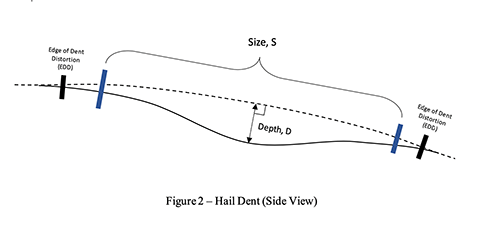## 2. Terminology

This section contains brief definitions (in alphabetical order) of the terms and acronyms that are graphically shown in Figure 1 and Figure 2.

Depth: The maximum distance, D, from the Normalized Surface to the profile of a dent. This quantity will not be discussed further, except to say that depth is generally not used in North American markets and is used in some European markets.

Extent of Dent Distortion (EDD): The maximum distance between any two edge points along the extent curve. The Extent of a dent can be determined visually (EDDV) or automatically (EDDA). The extent of distortion is not needed to measure the size of a dent and is mentioned in this procedure for completeness only. Refer to other Quidient procedures for steps used to visually measure EDDV.

Normalized Surface: The undented surface of the automobile panel, sometimes called the “Class A” surface because reflections are smooth and appealing to the human eye. The normalized surface can be determined using a CAD model of the vehicle or can be determined by measuring the undisturbed surface around a dent and interpolating using Class A surface (smoothness) considerations.

Repeatability: A measurement of variability obtained by taking successive measurements multiple times by a same person using the same instrument or technique.

Reproducibility: A measurement of variability obtained by taking successive measurements of the same entity, done using same equipment but by a different person.

Size of a dent, S: referring to Figure 1 and Figure 2 above, the size of a dent, S, is a diameter determined visually, SV, or automatically, SA, as further defined below:

SV (Visually Determined Size) is the diameter that one of more dent assessment experts associate with a dent based on their training and experience. Dent size can be expressed in units of coin size or units of distance (such as millimeters). Many (but not all) experts say that the size of a vehicle dent is the largest diameter of the region that must be worked by a repair professional to repair a dent. Refer to reference [A] for one dent size determination training video.

SA (Automatically Determined Size) is the diameter of a dent recorded one or more times by a coordinate measuring machine (CMM). As of the publication date of this procedure, there is no broadly accepted method for determining SA automatically. So, SA is determined using a hybrid analytic / machine learning process as follows:

Referring to Figure 3, the reference size, SR, of a dent is taken to be the six-sigma breadth of dent profile deviations measured by such a CMM, where the deviations are the distances between the Normalized surface and the actual surface at points in the dent.

The automatically determined dent size, SA, is defined to be k (a learning coefficient, defined in Section 3 below, used to train the CMM to measure like a dent assessment expert) times the reference size, SR, according to the equation below: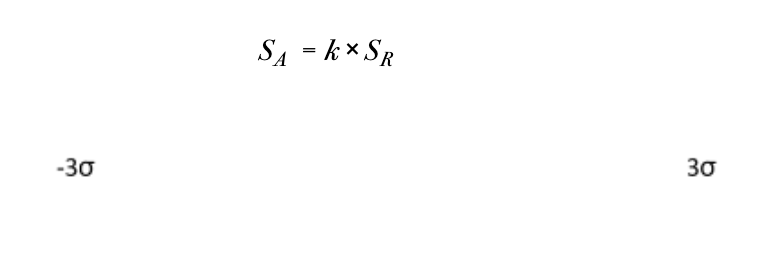Note that the SR, can be defined in various ways. The k value will adjust accordingly to train the CMM to simulate expert sizing.

## 3. Equations

The equations in this section reference characteristics shown in Figure 1 and Figure 2.

Mean

The average value of individual measurements.Deviation

Deviation from Measurement is the deviation of individual measurement from the meanMaximum Deviation

Max deviation is the max deviation between the successive measurements.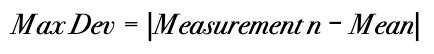Standard Deviation

Standard deviation is the value of Std Dev between the two measurements.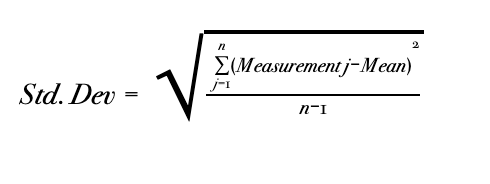## 4. Equipment

The items described in Table 1may be used in this procedure:

Table 1 – Equipment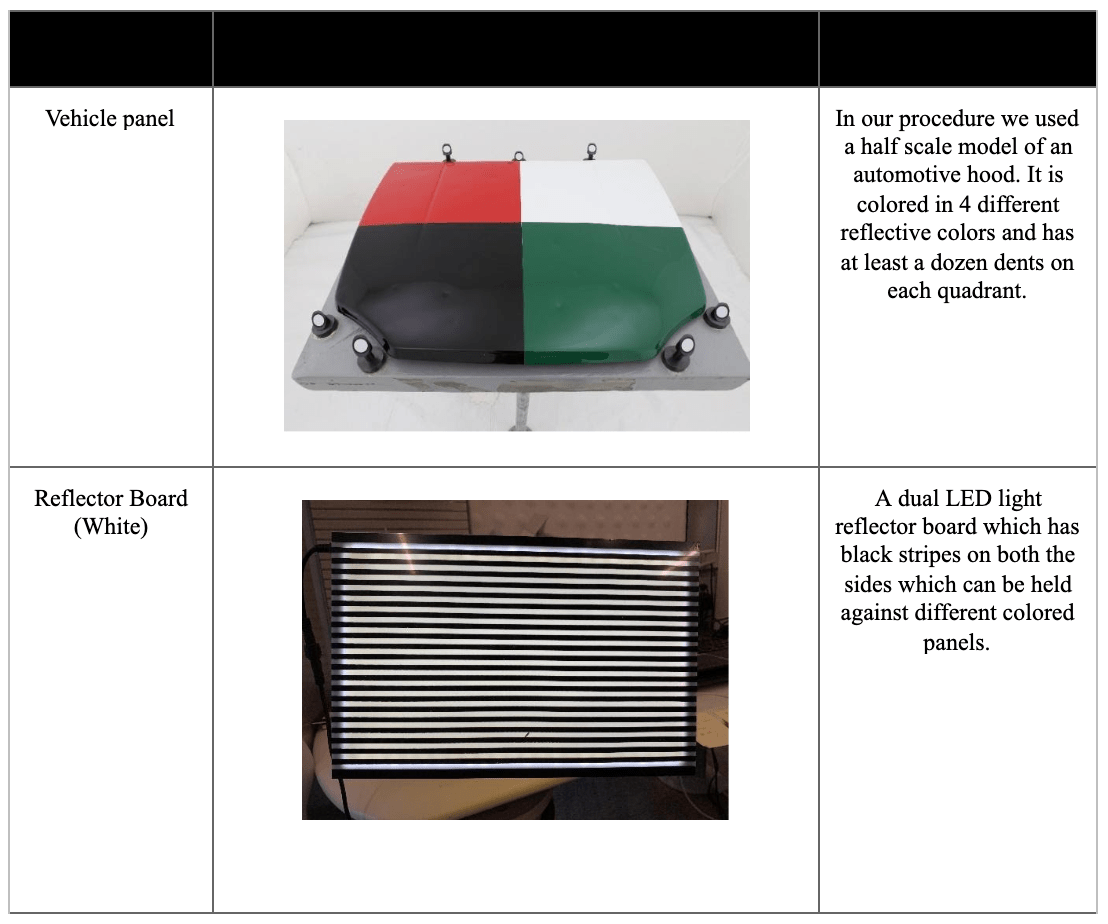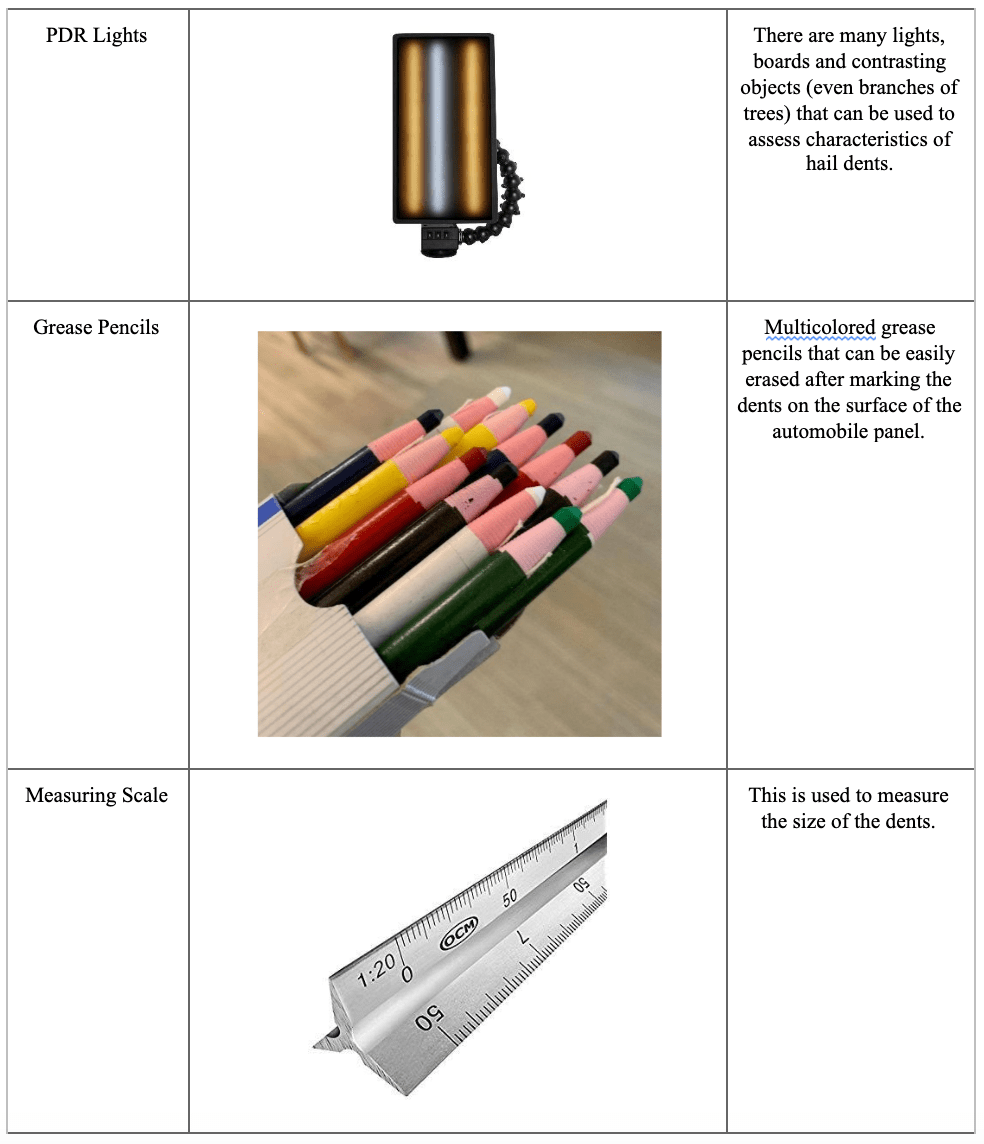## 5-1. Measuring SV, the Visually Determined Size of a Dent

1. Using the equipment and procedures required by the governing authority, measure the size of the dent. The procedure might specify, for example, equipment and lighting setup. Some governing authorities may require that the dent size be expressed in units of distance. Others may require that it be expressed in units of coinage.
2. Use the following table for classifying dent size if not otherwise specified by the governing authority.

This site uses Akismet to reduce spam. Learn how your comment data is processed.